No matter which 3rd-grade learning outcome you are teaching, there is a math game for you! 3rd-graders will not only find these math games fun and engaging, but games are also a great way to practice math skills.

The 3rd-grade is the start of multiplication, fractions, and more complex number properties.

### 1. DragonBox NumbersDragonBox is a unique app that allows 3rd-graders to deepen their intuitive understanding of numbers and algebra. The fundamentals are hidden within clever drawings and cards. The intuitive problem-solving games allow kids to have fun while learning.

Dragonbox

### 2. Math TangoMath Tango has a unique, classroom-tested combination of puzzle and world-building activities. 3rd-graders will enjoy building up their maths fluency in addition, subtraction, multiplication, and division while going on missions.

## 3. Subtraction Mountain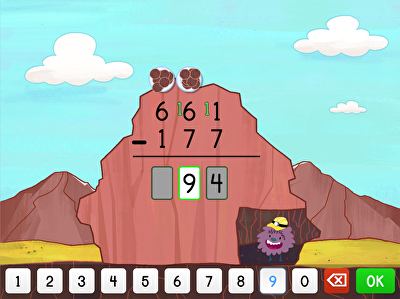In Subtraction Mountain, students help a friendly miner with three-digit subtraction. This game is good for practicing subtraction. Students might find it useful to think of the concept of subtraction as a movement downwards.

Subtraction Mountain

### 4. Professor BeardoHelp Professor Beardo create a magic beard-growth potion in this fun online game. Not only will students practice their addition skills, but it will reinforce the use of place-value in addition.

Professor Beardo3rd-graders are introduced to the commutative, associative, and identity properties of addition in this great addition game.

mathgames.com

### 6. Can You Make It?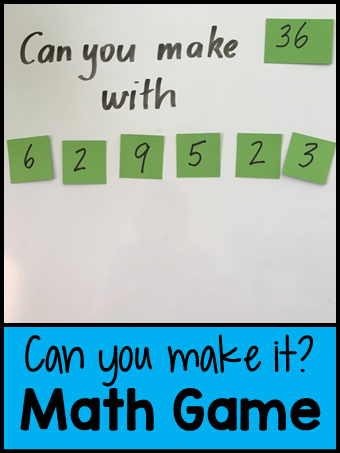Give students a set of numbers and a target number. See how many different ways they can use the numbers to get to the target number.

## Multiplication and Division

### 7. 3D Multiplication with Legos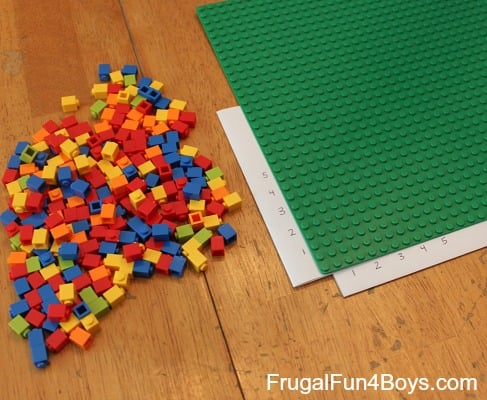Using Lego to build towers introduced students to the idea of grouping, multiplication, division, and the commutative property all at the same time!

frugalfun4boys.com

### 8. Candy Shop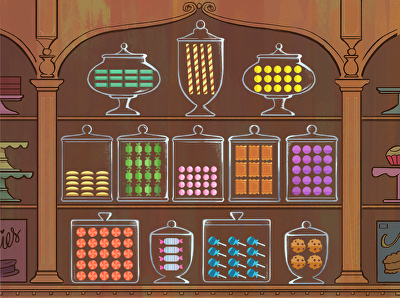Candy Shop makes multiplication a little sweeter (haha, get it?) by getting 3rd-graders to find the candy jars containing the correct multiplication array. In the process, they will gain an understanding of counting rows and columns to represent multiplication.

Candy Shop

Count Your Dots is a way of strengthening both the concept of multiplication as an array and multiplication as repeated addition. Using a deck of playing cards, each player flips two cards. You then draw horizontal lines that represent the number on your first card, and vertical lines to represent the number on your second card. On this gird, you make a dot where the lines join. Each player counts the dots, and the person with the most dots keeps all of the cards.

teachbesideme.com

### 10. Mathgames.comMathgames.com is a great online platform for practicing maths skills. This multiplication game gives students a chance to practice multiplication and get instant feedback. Their division game encourages students to think of division as a function by creating an input-output rule for division.

mathgames.com

### 11. Flip Dominoes and Multiply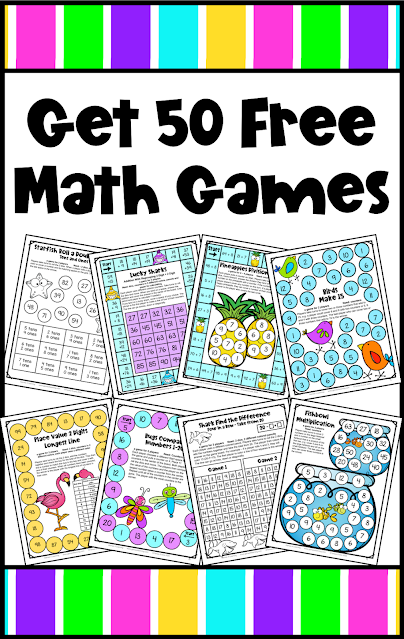This is a good way to help your 3rd-graders memorize multiplication facts. Each player flips a domino and multiplies the two numbers. The one with the highest product gets both dominoes.

fungames4learning.com

### 12. Divide and Conquer Division PairsAnother variation on Go Fish, but with division. Instead of matching cards according to suite or number, students form pairs by identifying two cards in which one can divide evenly into the other. For instance, 8 and 2 are a pair, since 8 ÷ 2 = 4.

cuppacocoa.com

## Fractions

### 13. Paper Fortune TellerAfter folding the traditional paper fortune teller, you can add your own math facts to the sections. For the fraction game, the first layer represents circles broken into fractions. The next level of flaps includes decimal numbers, and students have to figure out which ‘flap’ matches the circle. The last layer has a bar that students have to color using their fingers.

kidsactivitiesblog.com

### 14. Gem Mining Fraction Conversion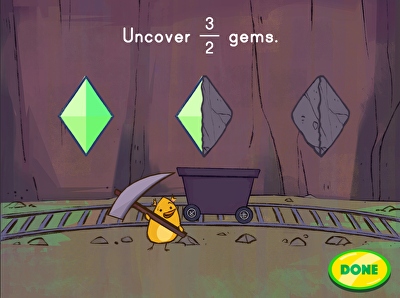Help our little underground gopher friend mine jewel fractions in this game about mining fractions.

education.com

### 15. Seashell Fractions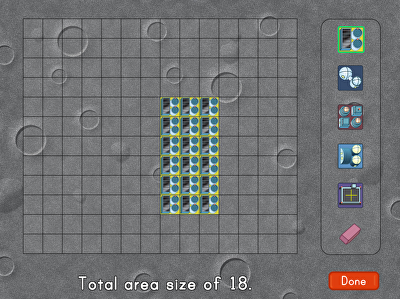This game about collecting seashell fractions gives students practice with identifying fractions in different contexts.

education.com

### 16. Using Lego Bricks to Create Fractions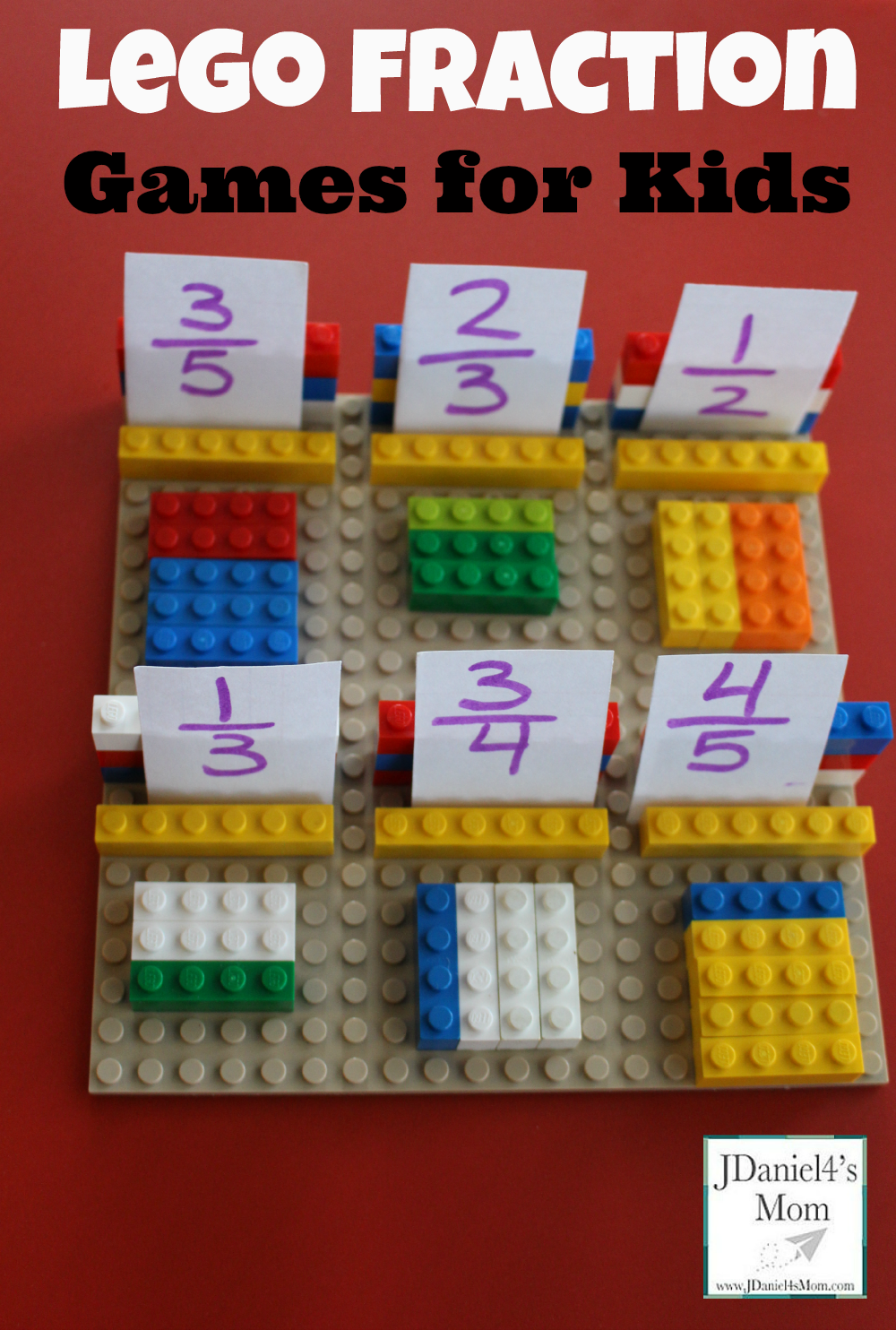Using Lego Bricks to create fractions is a great way of getting 3rd- graders to consider which part of the whole each brick represents.

jdaniel4smom.com

### 17. Fraction Match Game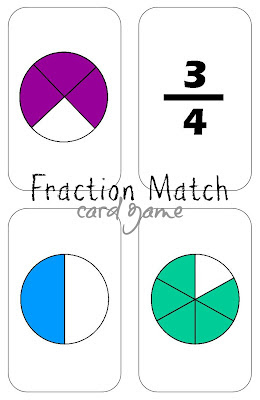Download the fraction match flashcards to play a modified version of Go Fish or Snap.

### 18. Comparing Fractions with Like Denominators: Space Voyage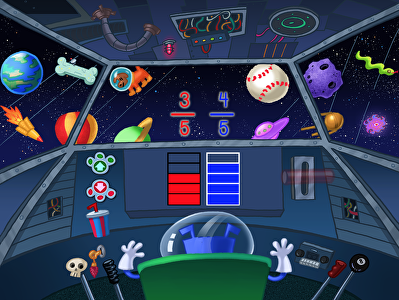Use the context of space travels to develop fluency in comparing fractions with like denominators.

education.com

### 19. Jumpy: Equivalent Fractions3rd-graders will practice identifying equivalent fractions while jumping from object to object on their way to the party.

education.com

### 20. Fraction Match-UpThis free printout gives your 3rd-graders a chance to make matches between the pictures and the fractions they represent. The trading element of this game reinforces the equivalence of the fractions.

### 21. Fraction War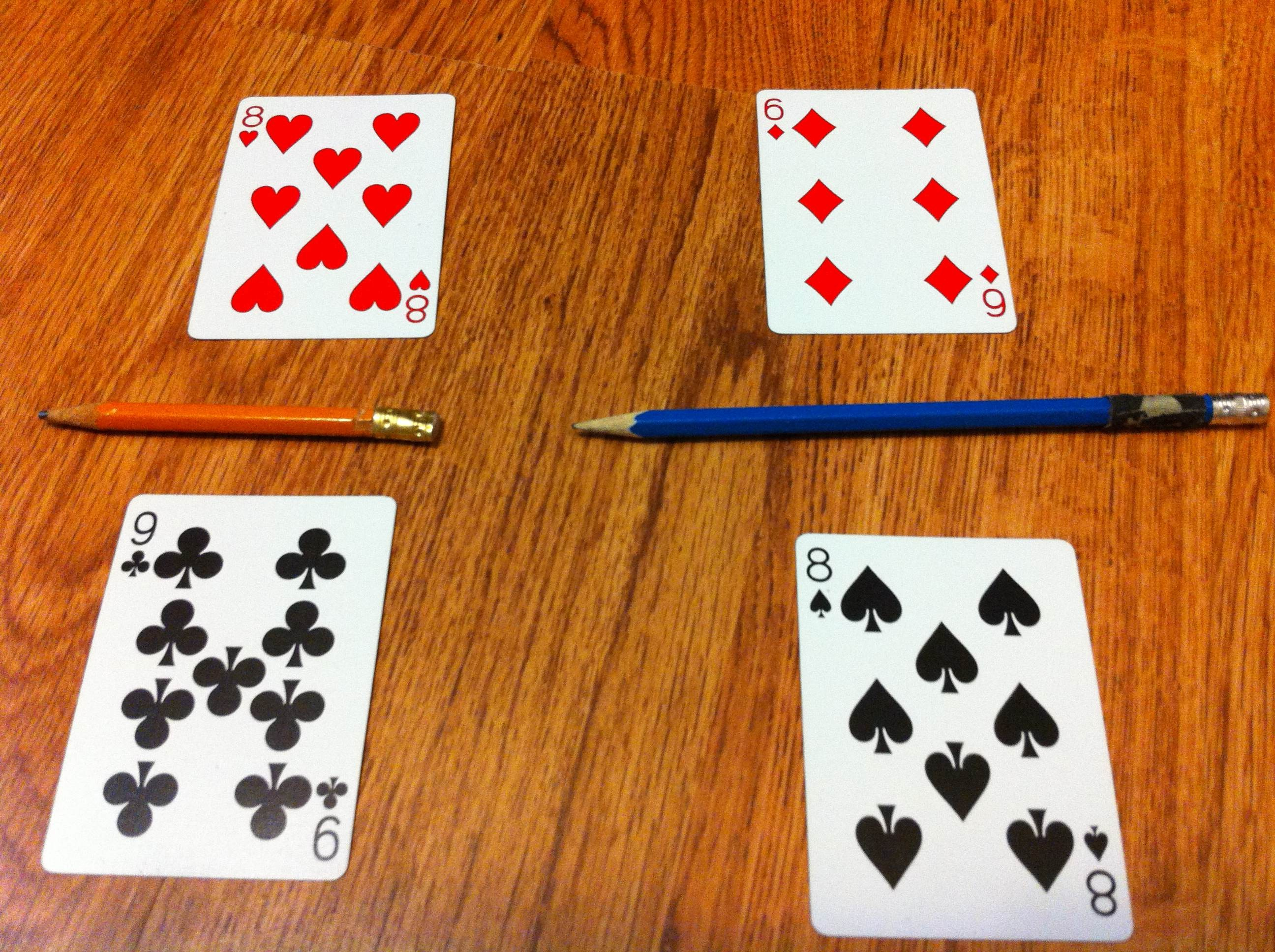Fraction War is a great game for your more advanced 3rd-graders. Each player flips two cards and they lay them out as a fraction. It can be useful to place a pencil between the top and the bottom card to separate the numerator from the denominator. The students decide which fraction is the greatest, and the winner keeps all the cards. Comparing the fractions with online denominators gets a little tricky, but if the students plot them on a fraction number line first, they’ll be practicing two skills at once.

mathfilefoldergames.com

## Other Topics

### 22. Match up LEGO bricks to tell timeWrite times in a variety of ways on Lego bricks and have students see how quickly they can match them up.

scholastic.com

### 23. Array CaptureUsing two dice, students take turns drawing arrays that represent the area of their throw. The student that fills us most of the page wins.

jillianstarrteaching.com

## 3rd-Grade Math Games for Every Standard

Whether you are teaching complex properties of numbers, multiplication, and division, or introducing your 3rd-graders to fractions, we’ve got a math game for you! Remember that we are trying to use games to improve learning, not just to fill time. You want your 3rd-graders to be engaged and having fun. But you need to do this in a way that supports your teaching and supports their learning.